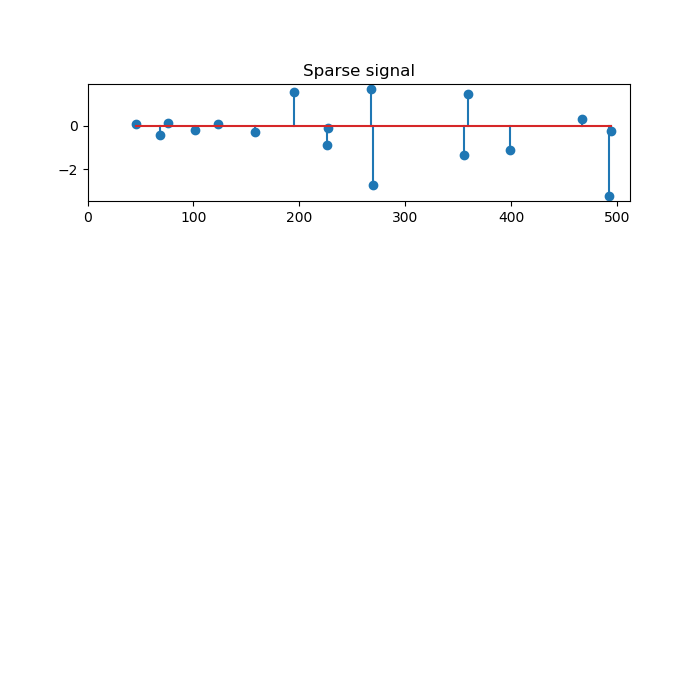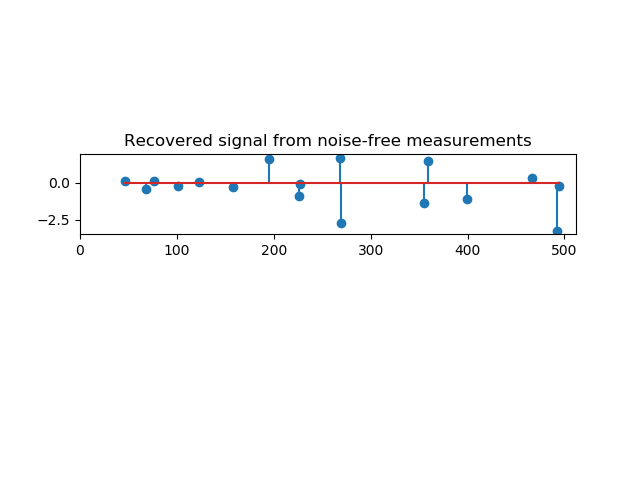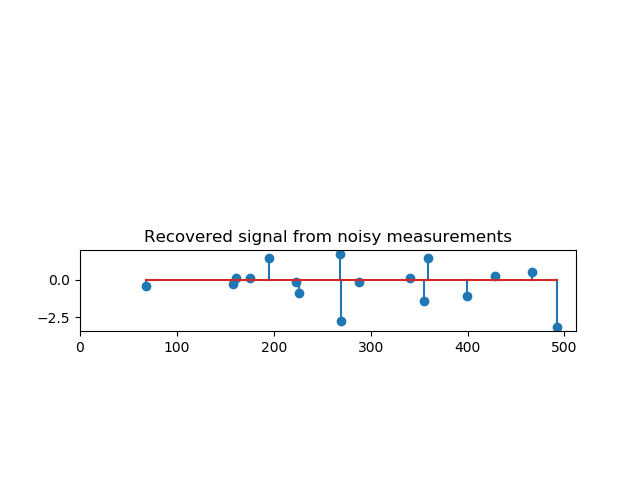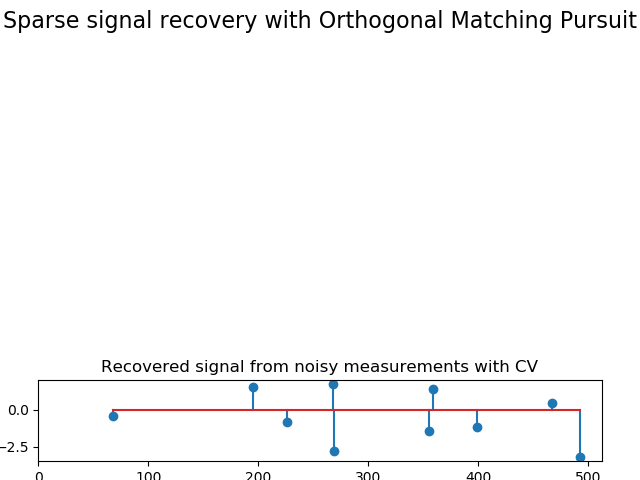# Orthogonal Matching Pursuit¶

Using orthogonal matching pursuit for recovering a sparse signal from a noisy measurement encoded with a dictionary

print(__doc__)

import matplotlib.pyplot as plt
import numpy as np
from sklearn.linear_model import OrthogonalMatchingPursuit
from sklearn.linear_model import OrthogonalMatchingPursuitCV
from sklearn.datasets import make_sparse_coded_signal

n_components, n_features = 512, 100
n_nonzero_coefs = 17

# generate the data
###################

# y = Xw
# |x|_0 = n_nonzero_coefs

y, X, w = make_sparse_coded_signal(n_samples=1,
n_components=n_components,
n_features=n_features,
n_nonzero_coefs=n_nonzero_coefs,
random_state=0)

idx, = w.nonzero()

# distort the clean signal


Out:



y_noisy = y + 0.05 * np.random.randn(len(y))

# plot the sparse signal

plt.figure(figsize=(7, 7))
plt.subplot(4, 1, 1)
plt.xlim(0, 512)
plt.title("Sparse signal")
plt.stem(idx, w[idx])

# plot the noise-free reconstructionOut:

/home/circleci/project/examples/linear_model/plot_omp.py:44: UserWarning: In Matplotlib 3.3 individual lines on a stem plot will be added as a LineCollection instead of individual lines. This significantly improves the performance of a stem plot. To remove this warning and switch to the new behaviour, set the "use_line_collection" keyword argument to True.
plt.stem(idx, w[idx])

omp = OrthogonalMatchingPursuit(n_nonzero_coefs=n_nonzero_coefs)
omp.fit(X, y)
coef = omp.coef_
idx_r, = coef.nonzero()
plt.subplot(4, 1, 2)
plt.xlim(0, 512)
plt.title("Recovered signal from noise-free measurements")
plt.stem(idx_r, coef[idx_r])

# plot the noisy reconstructionOut:

/home/circleci/project/examples/linear_model/plot_omp.py:56: UserWarning: In Matplotlib 3.3 individual lines on a stem plot will be added as a LineCollection instead of individual lines. This significantly improves the performance of a stem plot. To remove this warning and switch to the new behaviour, set the "use_line_collection" keyword argument to True.
plt.stem(idx_r, coef[idx_r])

omp.fit(X, y_noisy)
coef = omp.coef_
idx_r, = coef.nonzero()
plt.subplot(4, 1, 3)
plt.xlim(0, 512)
plt.title("Recovered signal from noisy measurements")
plt.stem(idx_r, coef[idx_r])

# plot the noisy reconstruction with number of non-zeros set by CVOut:

/home/circleci/project/examples/linear_model/plot_omp.py:66: UserWarning: In Matplotlib 3.3 individual lines on a stem plot will be added as a LineCollection instead of individual lines. This significantly improves the performance of a stem plot. To remove this warning and switch to the new behaviour, set the "use_line_collection" keyword argument to True.
plt.stem(idx_r, coef[idx_r])

omp_cv = OrthogonalMatchingPursuitCV(cv=5)
omp_cv.fit(X, y_noisy)
coef = omp_cv.coef_
idx_r, = coef.nonzero()
plt.subplot(4, 1, 4)
plt.xlim(0, 512)
plt.title("Recovered signal from noisy measurements with CV")
plt.stem(idx_r, coef[idx_r])

plt.subplots_adjust(0.06, 0.04, 0.94, 0.90, 0.20, 0.38)
plt.suptitle('Sparse signal recovery with Orthogonal Matching Pursuit',
fontsize=16)
plt.show()Out:

/home/circleci/project/examples/linear_model/plot_omp.py:77: UserWarning: In Matplotlib 3.3 individual lines on a stem plot will be added as a LineCollection instead of individual lines. This significantly improves the performance of a stem plot. To remove this warning and switch to the new behaviour, set the "use_line_collection" keyword argument to True.
plt.stem(idx_r, coef[idx_r])


Total running time of the script: ( 0 minutes 0.636 seconds)

Gallery generated by Sphinx-Gallery